Solving Pair of Linear Inequalities

Chapter 5 Class 11 Linear Inequalities
Serial order wise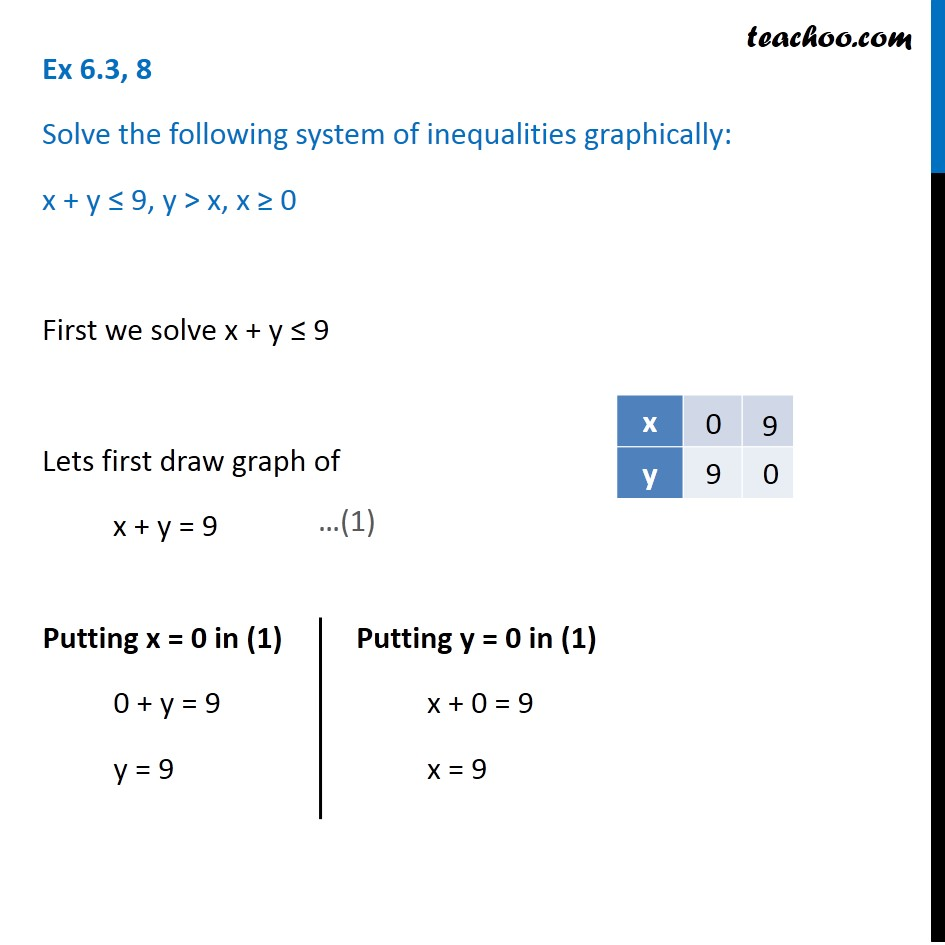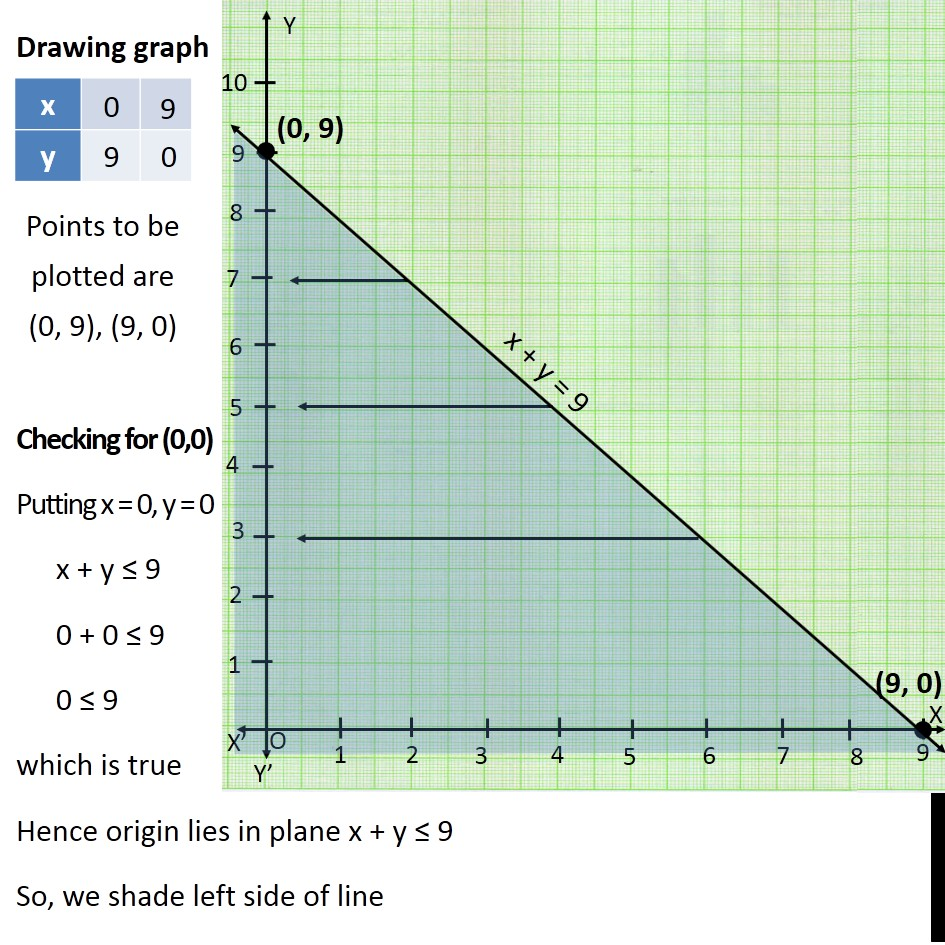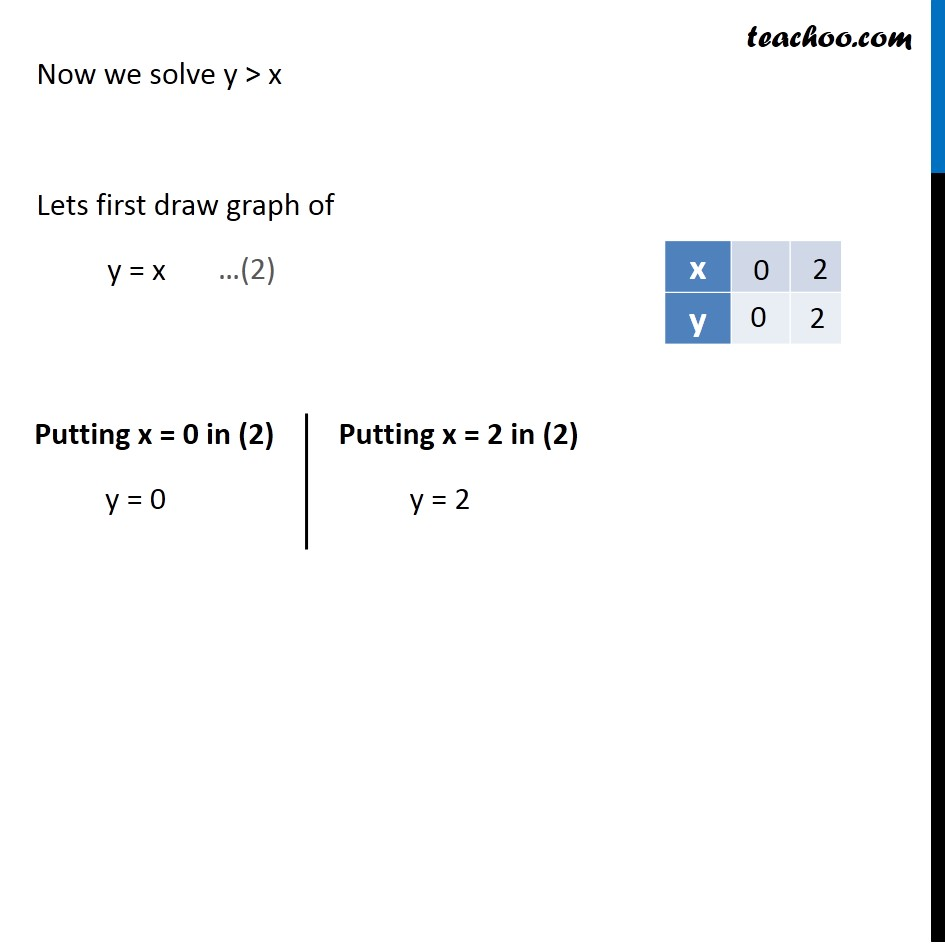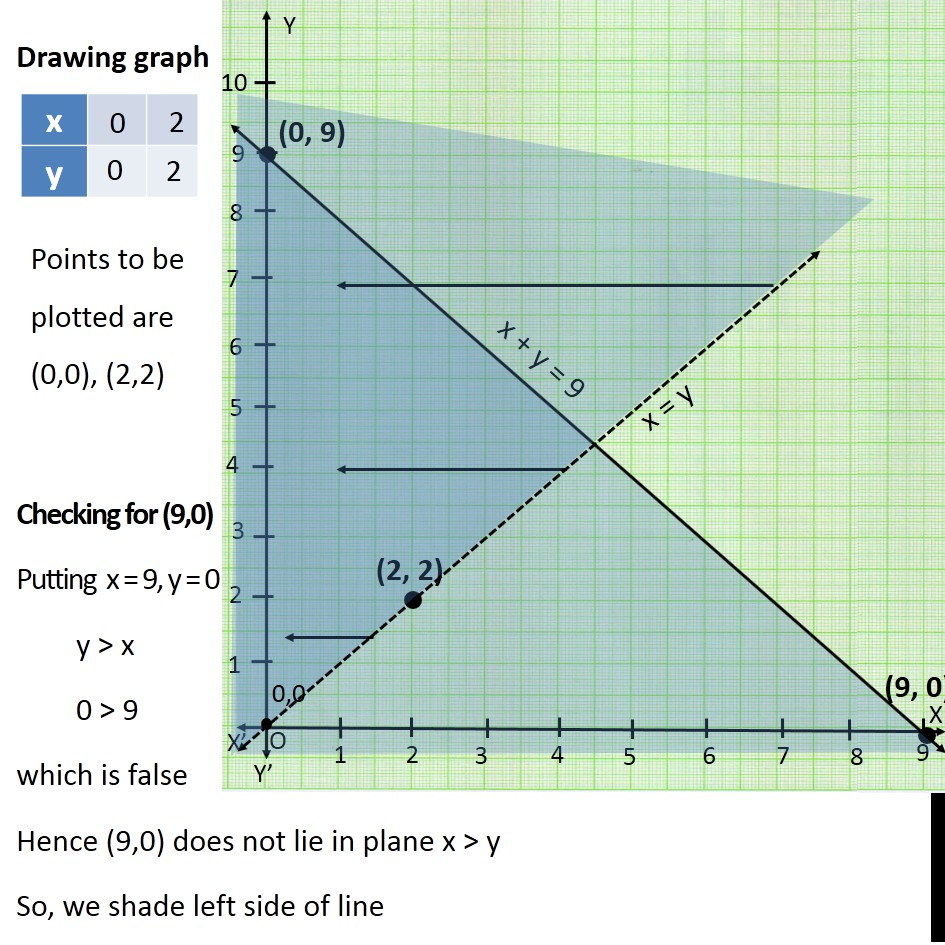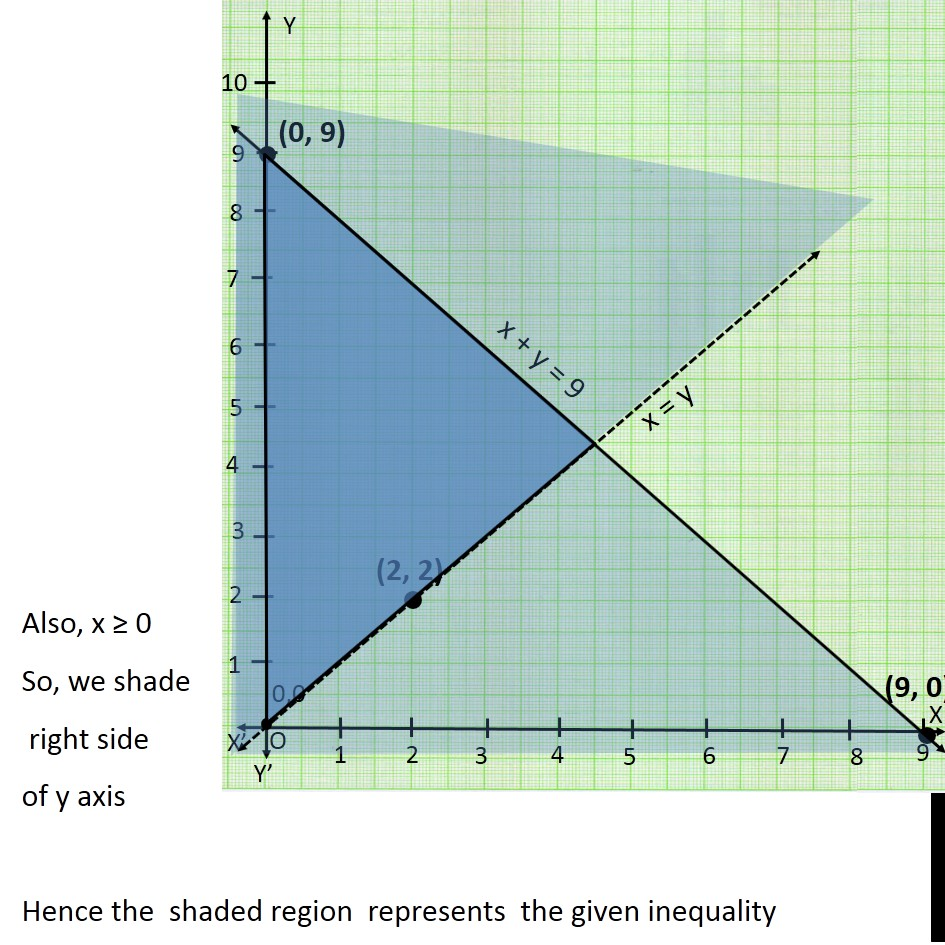Learn in your speed, with individual attention - Teachoo Maths 1-on-1 Class

### Transcript

Question 8 Solve the following system of inequalities graphically: x + y ≤ 9, y > x, x ≥ 0 First we solve x + y ≤ 9 Lets first draw graph of x + y = 9 Putting x = 0 in (1) 0 + y = 9 y = 9 Putting y = 0 in (1) x + 0 = 9 x = 9 Points to be plotted are (0, 9), (9, 0) Drawing graph Checking for (0,0) Putting x = 0, y = 0 x + y ≤ 9 0 + 0 ≤ 9 0 ≤ 9 which is true Hence origin lies in plane x + y ≤ 9 So, we shade left side of line Now we solve y > x Lets first draw graph of y = x Putting x = 0 in (2) y = 0 Putting x = 2 in (2) y = 2 Points to be plotted are (0,0), (2,2) Drawing graph Checking for (9,0) Putting x = 9, y = 0 y > x 0 > 9 which is false Hence (9,0) does not lie in plane x > y So, we shade left side of line Also, x ≥ 0 So, we shade right side of y axis Hence the shaded region represents the given inequality